Tamil Nadu Board of Secondary EducationHSC Science Class 12th

# Tamil Nadu Board Samacheer Kalvi solutions for Class 12th Physics Volume 1 and 2 Answers Guide chapter 2 - Current Electricity [Latest edition]

#### Chapters## Chapter 2: Current Electricity

Evaluation
Evaluation [Pages 118 - 123]

### Tamil Nadu Board Samacheer Kalvi solutions for Class 12th Physics Volume 1 and 2 Answers Guide Chapter 2 Current Electricity Evaluation [Pages 118 - 123]

#### Multiple Choice Questions

Evaluation | Q I. 1. | Page 118

The following graph shows current versus voltage values of some unknown conductor. What is the resistance of this conductor?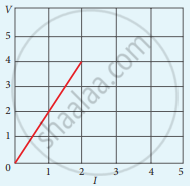• 2 ohm

• 4 ohm

• 8 ohm

• 1 ohm

Evaluation | Q I. 2. | Page 118

A wire of resistance 2 ohms per meter is bent to form a circle of radius 1m. The equivalent resistance between its two diametrically opposite points, A and B as shown in the figure is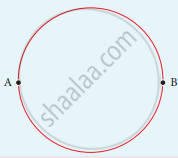• π Ω

• π/2 Ω

• 2 π Ω

• π/4 Ω

Evaluation | Q I. 3. | Page 118

A toaster operating at 240 V has a resistance of 120 Ω. Its power is ______.

• 400 W

• 2 W

• 480 W

• 240 W

Evaluation | Q I. 4. | Page 118

A carbon resistor of (47 ± 4.7 ) k Ω to be marked with rings of different colours for its identification. The colour code sequence will be ______.

• Yellow – Green – Violet – Gold

• Yellow – Violet – Orange – Silver

• Violet – Yellow – Orange – Silver

• Green – Orange – Violet - Gold

Evaluation | Q I. 5. | Page 118

What is the value of resistance of the following resistor?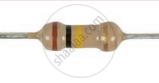• 100 k Ω

• 10 k Ω

• 1 k Ω

• 1000 k Ω

Evaluation | Q I. 6. | Page 118

Two wires of A and B with circular cross-section are made up of the same material with equal lengths. Suppose RA = 3RB, then what is the ratio of radius of wire A to that of B?

• 3

• sqrt3

• 1/sqrt3

• 1/3

Evaluation | Q I. 7. | Page 118

A wire connected to a power supply of 230 V has power dissipation P1. Suppose the wire is cut into two equal pieces and connected parallel to the same power supply. In this case, power dissipation is P2. The ratio of "P"_2/"P"_1 is

• 1

• 2

• 3

• 4

Evaluation | Q I. 8. | Page 119

In India electricity is supplied for domestic use at 220 V. It is supplied at 110 V in USA. If the resistance of a 60W bulb for use in India is R, the resistance of a 60W bulb for use in USA will be

• R

• 2R

• "R"/4

• "R"/2

Evaluation | Q I. 9. | Page 118

In a large building, there are 15 bulbs of 40 W, 5 bulbs of 100 W, 5 fans of 80 W, and 1 heater of 1 kW are connected. The voltage of electric mains is 220 V. The minimum capacity of the main fuse of the building will be

• 14 A

• 8 A

• 10 A

• 12 A

Evaluation | Q I. 10. | Page 119

There is a current of 1.0 A in the circuit shown below. What is the resistance of P?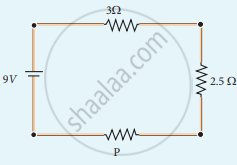• 1.5 Ω

• 2.5 Ω

• 3.5 Ω

• 4.5 Ω

Evaluation | Q I. 11. | Page 119

What is the current out of the battery?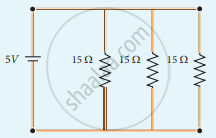• 1A

• 2A

• 3A

• 4A

Evaluation | Q I. 12. | Page 119

The temperature deficient of resistance of a wire is 0.00125 per°C At 20°C, its resistance is 1Ω. The resistance of the wire will be 2Ω at

• 800°C

• 700°C

• 850°C

• 820°C

Evaluation | Q I. 13. | Page 119

The internal resistance of a 2.1 V cell which gives a current of 0.2 A through a resistance of 10Ω is ______.

• 0.2 Ω

• 0.5 Ω

• 0.8 Ω

• 1.0 Ω

Evaluation | Q I. 14. | Page 119

A piece of copper and another of germanium are cooled from room temperature to 80 K. The resistance of ______.

• each of them increases

• each of them decreases

• copper increases and germanium decreases

• copper decreases and germanium increases

Evaluation | Q I. 15. | Page 119

In Joule’s heating law, when I and t are constant, if the H is taken along the y axis and I2 along the x axis, the graph is ______.

• straight line

• parabola

• circle

• ellipse

Evaluation | Q II. 1. | Page 119

Why current is a scalar?

Evaluation | Q II. 2. | Page 119

Define Current density.

Evaluation | Q II. 3. | Page 119

Distinguish between drift velocity and mobility.

Evaluation | Q II. 4. | Page 120

State microscopic form of Ohm’s law.

Evaluation | Q II. 5. | Page 120

State macroscopic form of Ohm’s law.

Evaluation | Q II. 6. | Page 120

What is ohmic device?

Evaluation | Q II. 6. | Page 120

What is non ohmic device?

Evaluation | Q II. 7. | Page 120

Define electrical resistivity.

Evaluation | Q II. 8. | Page 120

Define temperature coefficient of resistance.

Evaluation | Q II. 9. | Page 120

Write a short note on superconductors?

Evaluation | Q II. 10. | Page 120

What is electric power?

Evaluation | Q II. 10 | Page 120

What is electric energy?

Evaluation | Q II. 11. | Page 120

Derive the expression for power P = VI in electrical circuit.

Evaluation | Q II. 12. | Page 120

Write down the various forms of expression for power in electrical circuit.

Evaluation | Q II. 13. | Page 120

State Kirchhoff’s current rule.

Evaluation | Q II. 14. | Page 120

State Kirchhoff ’s voltage rule.

Evaluation | Q II. 15. | Page 120

State the principle of potentiometer.

Evaluation | Q II. 16. | Page 120

What do you mean by internal resistance of a cell?

Evaluation | Q II. 17. | Page 120

State Joule’s law of heating.

Evaluation | Q II. 18. | Page 120

What is Seebeck effect?

Evaluation | Q II. 19. | Page 120

What is Thomson effect?

Evaluation | Q II. 20. | Page 120

What is Peltier effect?

Evaluation | Q II. 21. | Page 120

State the applications of Seebeck effect.

Evaluation | Q III. 1. | Page 120

Describe the microscopic model of current and obtain general form of Ohm’s law.

Evaluation | Q III. 2. | Page 120

Obtain the macroscopic form of Ohm’s law from its microscopic form and discuss its limitation.

Evaluation | Q III. 3. | Page 120

Explain the equivalent resistance of a series resistor network.

Evaluation | Q III. 3. | Page 120

Explain the equivalent resistance of a parallel resistor network.

Evaluation | Q III. 4. | Page 120

Explain the determination of the internal resistance of a cell using voltmeter.

Evaluation | Q III. 5. | Page 120

State and explain Kirchhoff’s rules.

Evaluation | Q III. 6. | Page 120

Obtain the condition for bridge balance in Wheatstone’s bridge.

Evaluation | Q III. 7. | Page 120

Explain the determination of unknown resistance using meter bridge.

Evaluation | Q III. 8. | Page 120

How the emf of two cells are compared using potentiometer?

#### Numerical problems

Evaluation | Q IV. 1. | Page 120

The following graphs represent the current versus voltage and voltage versus current for the six conductors A, B, C, D, E and F. Which conductor has least resistance and which has maximum resistance?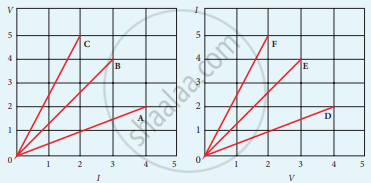Evaluation | Q IV. 2. | Page 120

Lightning is a very good example of a natural current. In typical lightning, there is 109 J energy transfer across the potential difference of 5 × 107 V during a time interval of 0.2 s. Using this information, estimate the following quantities:

1. the total amount of charge transferred between cloud and ground
2. the current in the lightning bolt
3. the power delivered in 0.2 s.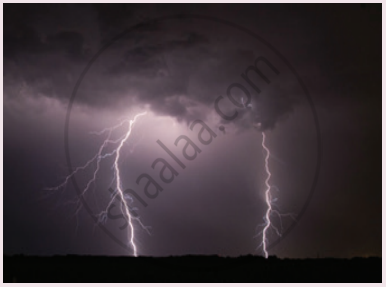Evaluation | Q IV. 3. | Page 121

A copper wire of 10-6 m2 area of cross-section, carries a current of 2 A. If the number of electrons per cubic meter is 8 × 1028, calculate the current density and average drift velocity.

Evaluation | Q IV. 4. | Page 121

The resistance of a nichrome wire at 0°C is 10Ω. If its temperature coefficient of resistivity of nichrome is 0.004/ °C, find its resistance of the wire at boiling point of water. Comment on the result.

Evaluation | Q IV. 5. | Page 121

The rod given in the figure is made up of two different materials.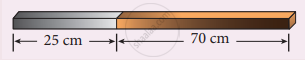Both have square cross-sections of 3 mm side. The resistivity of the first material is 4 x 10-3 Ω.m and it is 25 cm long while the second material has a resistivity of 5 x 10-3 Ω.m and is of 70 cm long. What is the resistivity of rod between its ends?

Evaluation | Q IV. 6. | Page 121

Three identical lamps each having a resistance R are connected to the battery of emf as shown in the figure.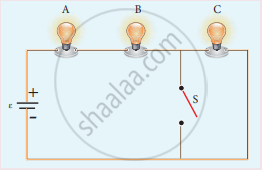Suddenly the switch S is closed.

1. Calculate the current in the circuit when S is open and closed.
2. What happens to the intensities of the bulbs A, B and C.
3. Calculate the voltage across the three bulbs when S is open and closed.
4. Calculate the power delivered to the circuit when S is opened and closed.
5. Does the power delivered to the circuit decrease, increase or remain same?
Evaluation | Q IV. 7. | Page 122

An electronics hobbyist is building a radio which requires 150 Ω in her circuit, but she has only 220 Ω, 79 Ω, and 92 Ω resistors available. How can she connect the available resistors to get the desired value of resistance?

Evaluation | Q IV. 8. | Page 122

A cell supplies a current of 0.9 A through a 2 Ω resistor and a current of 0.3 A through a 7 Ω resistor. Calculate the internal resistance of the cell.

Evaluation | Q IV. 9. | Page 122

Calculate the currents in the following circuit.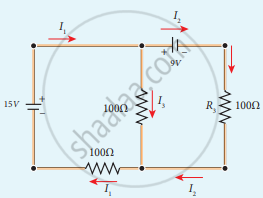Evaluation | Q IV. 10. | Page 122

A potentiometer wire has a length of 4 m and resistance of 20 Ω. It is connected in series with resistance of 2980 Ω and a cell of emf 4 V. Calculate the potential along the wire.

Evaluation | Q IV. 11. | Page 122

Determine the current flowing through the galvanometer (G) as shown in the figure.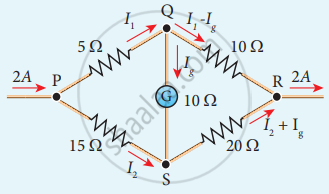Evaluation | Q IV. 12. | Page 122

Two cells each of 5V are connected in series across an 8Ω resistor and three parallel resistors of 4Ω, 6Ω, and 12Ω. Draw a circuit diagram for the above arrangement. Calculate

1. the current drawn from the cell
2. current through each resistor
Evaluation | Q IV. 13. | Page 122

Four light bulbs P, Q, R, S are connected in a circuit of unknown arrangement. When each bulb is removed one at a time and replaced, the following behavior is observed.

 P Q R S P removed * on on on Q removed on * on off R removed off off * off S removed on off on *

Draw the circuit diagram for these bulbs.

Evaluation | Q IV. 14. | Page 123

In a potentiometer arrangement, a cell of emf 1.25 V gives a balance point at 35 cm length of the wire. If the cell is replaced by another cell and the balance point shifts to 63 cm, what is the emf of the second cell?

## Chapter 2: Current Electricity

Evaluation## Tamil Nadu Board Samacheer Kalvi solutions for Class 12th Physics Volume 1 and 2 Answers Guide chapter 2 - Current Electricity

Tamil Nadu Board Samacheer Kalvi solutions for Class 12th Physics Volume 1 and 2 Answers Guide chapter 2 (Current Electricity) include all questions with solution and detail explanation. This will clear students doubts about any question and improve application skills while preparing for board exams. The detailed, step-by-step solutions will help you understand the concepts better and clear your confusions, if any. Shaalaa.com has the Tamil Nadu Board of Secondary Education Class 12th Physics Volume 1 and 2 Answers Guide solutions in a manner that help students grasp basic concepts better and faster.

Further, we at Shaalaa.com provide such solutions so that students can prepare for written exams. Tamil Nadu Board Samacheer Kalvi textbook solutions can be a core help for self-study and acts as a perfect self-help guidance for students.

Concepts covered in Class 12th Physics Volume 1 and 2 Answers Guide chapter 2 Current Electricity are Ohm's Law (V = IR), Electric Current, Energy and Power in Electrical Circuits, Electric Cells and Batteries, Kirchhoff’s Rules, Heating Effect of Electric Current, Thermoelectric Effect.

Using Tamil Nadu Board Samacheer Kalvi Class 12th solutions Current Electricity exercise by students are an easy way to prepare for the exams, as they involve solutions arranged chapter-wise also page wise. The questions involved in Tamil Nadu Board Samacheer Kalvi Solutions are important questions that can be asked in the final exam. Maximum students of Tamil Nadu Board of Secondary Education Class 12th prefer Tamil Nadu Board Samacheer Kalvi Textbook Solutions to score more in exam.

Get the free view of chapter 2 Current Electricity Class 12th extra questions for Class 12th Physics Volume 1 and 2 Answers Guide and can use Shaalaa.com to keep it handy for your exam preparation